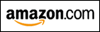INTEGRATION QUIZ #2

NAU STUDENTS:
This exercise gives you new problems to practice—ones requiring renaming before integrating.
Your in-class quiz will cover all the problems from the first integration quiz, together with these new problems.

In this exercise, all functions are assumed to have the required properties for a particular situation.
For example, any function in a denominator is assumed to be nonzero, any function inside a logarithm is assumed to be positive, and so on.

## SOMETIMES, YOU JUST NEED TO RENAME..

Sometimes, the function being integrated needs to be re-named, using basic algebra skills.
Perhaps you need to write a radical with a rational exponent.
Perhaps you need to use a distributive law.
Perhaps you need to FOIL something out.
Perhaps you need to use the fact that $\frac{A+B}C = \frac{A}{C} + \frac{B}{C}\,$.

### EXAMPLE:

$\displaystyle\int\frac{1}{\root 3\of x}\ dx = \int x^{-1/3}\ dx = \frac{x^{-\frac 13 + 1}}{-\frac13 + 1} + C = \frac{x^{2/3}}{2/3} + C = \frac 32 \root 3\of{x^2} + C$

If a problem starts off in radical form, then you should give your answer in radical form.
Recall that dividing by a fraction is the same as multiplying by its reciprocal: e.g., dividing by $\,\frac 23\,$ is the same as multiplying by $\,\frac 32\,$.
Also recall that for $\,n\ne -1\,$, $\,\int x^n\,dx = \frac{x^{n+1}}{n+1} + C\,$.

### EXAMPLE:

$\displaystyle\int \frac{x^3-1}{x}\ dx = \int \bigl(\frac{x^3}x - \frac 1x\bigr)\ dx = \int( x^2 - \frac 1x)\ dx = \frac{x^3}3 - \ln |x| + C$
Master the ideas from this section
by practicing the exercise at the bottom of this page.

On this exercise, you will not key in your answer.
However, you can check to see if your answer is correct.Is this web exercise helping you? I'm spending many hours creating it. Please help me to help you... just watch my 15-second video to see how easy it is to support my work, at absolutely no cost to you! (Really!) Thank you!